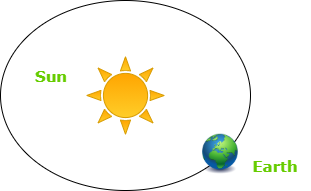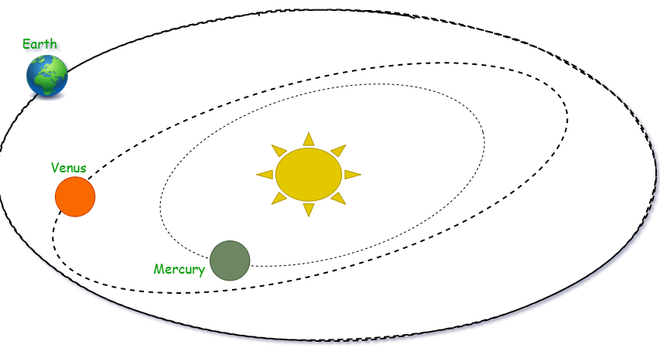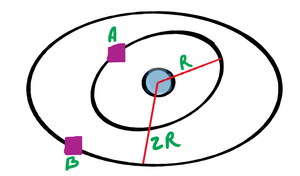Related Articles

# Newton’s Universal Law of Gravitation

• Last Updated : 07 Apr, 2021

Whether or not that apple actually landed on Isaac Newton’s head, as some stories would have it, this equation given by universal law of gravitation describes why everyone stays rooted to the ground, what locks the Earth in orbit around the sun, and to send men to the moon. It summarizes the idea that all the particles of matter in the universe attract each other through the force of gravity, Newton’s law tells how strong that attraction is. Let’s discuss how this law come to light and derived.

### What is Gravitation?

Gravitation is a force that states that every object on the earth or in space attracts each other. The gravitational force on the object depends on the mass of the object, the more the mass of the object more will be the force applied to it by the other objects. All objects which we could see are attracted by each other by some amount, like pen, eraser, planets, mobile phone, watch, fridge. Gravitational force is one of the non-contact forces with the Electromagnetic Force and the Nuclear Force.Earth rotating in its orbit around the sun as it is attracted by Sun’s gravity.

### Universal Law of Gravitation

Every object in the universe attract every other object by a force which is directly proportional to product of masses of both objects and inversely proportional to square of the distance in between them. This is known as Universal Law of Gravitation. This has very interesting history and lots of handwork and research of many scientists and mathematicians.

One day Isaac Newton was sitting under an apple tree in his college. An apple fell down on earth, and he thought why did this apple fell down, why this did not go upward. This curiosity strikes him hard, and he started working on it and find a mathematical solution for the apple to fall down. He gave a law for this, called “The Universal Law of Gravitation”.Representation for Universal Law of Gravitation

According to Newton’s universal law of gravitation the gravitational force that exist between the two masses say m1 and m2 is directly proportional to the product of their masses and inversely proportional to the square of the distance between the two masses i.e. d.

Mathematically, both the conditions can be interpreted as:

• F ∝ m1 × m2
• F ∝ 1 / d2

Combining the above two conditions, it is obtained as:

• F ∝ m1 × m2 / d2

Now, deducing the above condition to an equation. Remove the proportionality sign by introducing the constant of proportionality.Here, G is the constant of proportionality and known as the Gravitational constant equal to 6.67 × 10 −11 N⋅m2 / kg2.

### Significance of Universal Law of Gravitation

This universal law of Gravitation opened many paths for more research and to find the working of our universe with the existence of living organisms on the planets. Spacecraft uses this equation to calculate different results. Gravity is very important for us. It keeps us into the orbit to revolve around the Sun and get the sunlight and warmth. Gravity also hold the air and the earth’s atmosphere to it. Black hole are the place where you could see the power of the Gravitational force. Black hole engulf every thing even light and store every thing at a very small place in their tail called Singularity.

The force that binds us to the Earth:

All living and non-living things are on earth they don’t leave earth’s atmosphere and fall into outer space. Even though human have developed some technologies to penetrate this force and move out into space for the exploration. Gravitation is the force which bring us back on earth when we jump.Force on us by Earth

The motion of the Moon around Earth:

The giant-impact theory says that the moon was a part of earth and during earth’s early phases of creation it gets detached and started revolving around earth due to the gravitational force on the Moon. The Moon’s revolution time and rotation time are equal that is why we can only see it’s one side.The motion of Planets around Sun:

For a very long time it was a thing that people use to believe that sun revolve around sun. When Galileo Galilei said that earth revolve around the Sun, he was  ordered to come to the Holy Office for the trial, and he will be imprisoned and will remain in loneliness. Even the Sun also orbit around the Milky Way Galaxy. It takes 236 million years to complete an orbit. Our galaxy is a vast area of research.Motion of Planets around Sun

### Sample Problems

Problem 1: The mass of the earth is 6 × 1024 kg and that of the moon is 7.4 × 1022 kg. If the distance between the earth and the moon is 3.84 × 105 km, calculate the force exerted by the earth on the moon.

Solution:

Given that,

The mass of the earth, m1 is 6 × 1024 kg.

The mass of the moon, m2 is 7.4 × 1022 kg.

The distance between earth and moon, d is 3.84 × 105 km.

The formula to calculate the force exerted by the earth on the moon is:Hence, the force exerted by the earth on the moon is equal to 2.02 × 1020 N.

Problem 2: Your teacher is teaching you the law of gravitation 5 m far from you, if his Mass is 60 kg then what is the value of gravitational force exerted by you on him? (Hint: Take your weight by yourself).

Solution:

Given that,

The mass of the teacher, M is 60 kg.

The mass of the person, m is 70 kg.

The distance between them, d is 5 m.

The formula to calculate the gravitational force exerted by the person on the teacher is:Hence, the gravitational force exerted by the person on the teacher is 1.1 × 10-9 N.

Problem 3: A satellite A has twice the mass of satellite B. The satellite A orbit the planet with the half-radius of B satellite. How many times the force on A satellite in comparison to B satellite by earth?

Solution:

Consider the mass of B satellite as m and the mass of A satellite as 2m.

Now, to calculate gravitational force for both satellite divide both forces to obtain an equation as:Now divide the force on A by Force on B as:Satellites orbiting earth

Hence, the force on satellite A is 8 times the force on satellite B.

Problem 4: The Newton’s law of gravitation applies to:

a.  Small bodies only

b.  Plants only

c.  All bodies irrespective of their size

d.  For solar system

Solution:

The Newton’s law of gravitation is applicable to all bodies irrespective of their size.

Hence, Option c. is correct.

Problem 5: Consider two bodies in contact such that the distance between them is six times greater than the usual distance. Calculate how much the force changed.

Solution:

According to the Newton’s universal law, the gravitational force (F) is inversely proportional to the square of the distance (d) between the two objects in contact.

Mathematically:Consider the usual distance between the two bodies be x.

Then, the initial force is given as:Now, when the distance between the two bodies changed to 6x then the new force is given as:Now, divide the two forces as:Hence, the usual force is changed by 1/36 times the new force.

Attention reader! Don’t stop learning now. Join the First-Step-to-DSA Course for Class 9 to 12 students , specifically designed to introduce data structures and algorithms to the class 9 to 12 students

My Personal Notes arrow_drop_up